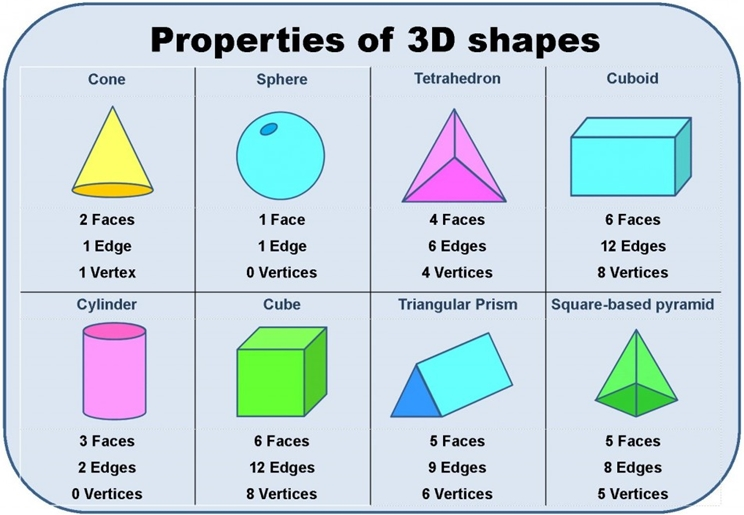# How many vertices does a cone have? Find outI recently saw an argument about how many vertices a cone has. First let me define what a vertex is. A vertex or vertices for plural, is the corner where two points meet. A flat 2D triangle for instance will have 3 vertices, but because a triangle is a 2 dimensional flat shape, it is much easier to determine where its points meet.

## How many vertices does a cone have?

When you have a two-dimensional shape like a cone, cube, cylinder, and rectangular prism, determining the amount of vertices becomes more complicated. You have to count the vertices from a three-dimensional angle while also keeping in mind, flat surfaces, make up most 3 D shapes. A cube for instance is just a bunch of 2D Squares joined together. While some have argued that a cone has no vertex because of the rounded meeting point, a cone actually had 1 Vertex as you can see in the picture below. The picture used below is from a popular math text-book used in schools across America.As you can see, even in 2 Dimensional form, a cone has 1 vertex. This does not change in it’s 3D state.

## Other 3D Shapes – Flat Surface and Vertices count

As a point of reference the following shapes have the corresponding amount of vertices

A cube had 6 flat surfaces and 8 vertices
A cone has 1 flat surface (the circle at the top) and technically 1 vertex
A rectangular prism has 6 flat surfaces and 8 vertices
A cylinder has 3 flat surfaces and no vertex

The cylinder is the only shape out of those listed we were able to prove categorically that it has no vertex.

## Picture Guide for Shapes and their Vertices

Also, I am sharing a picture guide that depicts the various shapes with the corresponding amount of vertices, faces and edges. You can print or save it for reference use.